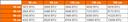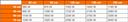British Thermal Units (BTU) is a measurement that can be used to determine how much heat an appliance can generate. In the UK, it is commonly referenced in kilowatts (kW). It describes the amount of energy that it would take to heat one pound of water (around one pint) by one degree fahrenheit.

When applied to radiators, it can be used to calculate whether the appliance is the right size for a room and whether it can heat the space efficiently. Alternatively, BTUs can also be found on appliances like air conditioning units. In this case, it describes how much heat the air conditioner could remove from the room.## How many BTU do I need?

The BTU requirements of a room depends on various factors, such as room size, insulation, type of glazing and ceiling height. For example, a room that has high ceilings will need more heat than a room that is of standard height.

You can also take the ideal room temperature for that space into account. For instance, you may want your bedroom to be a couple of degrees cooler than your living room. These factors are important to take into account as they could make a big difference to how cold or warm a room feels.

In some cases where the room is very large, two medium-sized radiators might be better than installing one large one. This is why you should calculate the BTU of a room before choosing a radiator.

## How many BTU for a bedroom?

The optimum bedroom temperature is between 16 and 18 ℃, so how many BTU do you need to reach these conditions?

First, use a tape measure to find out the height, width and length of your bedroom. Once you have these three measurements, multiply them together to get the cubic volume of the room.

For example, if your bedroom is 4.8 m in width, 4 m in length and 2.4 m in height, you should multiply these together to get a total figure of 46.08 metres cubed.

Now that you have the cubic volume, you should multiply this by 108 to calculate the BTU of your bedroom. In our above example, this would equal 4,976.64 BTU. However, if you have single glazing, you should instead multiply the cubic volume by 132, as the room will lose more heat. The samples above are for a typical approximation on heat loss for a UK bedroom.

## How many BTU do I need for my living room?

Just like we’ve done above, we’ll run through how you can find the BTU of your living room. This may slightly differ from a bedroom, as it’s likely that you’ll want this room to sit around two to three degrees warmer.

Once again, take the height, width and length of the room. If the room is an odd shape, don’t forget that you can separate it into multiple rectangles and then add together. To get the cubic volume, multiply the final three figures together.

For the bedroom, we multiplied this cubic volume by 108. Instead, if you have a living room with double glazing, multiply it by 135 to account for the additional warmth. The samples above are for a typical approximation on heat loss for a UK living room.

## How do I work out the BTU of my radiator?

Rather than working out the BTU of a room, you might want to find out the BTU of a radiator to determine if it’s big enough. You can calculate a rough estimate by seeing what kind of radiator it is and measuring the width and height.

The most common type of radiator is a double panel with fins, such as the one shown below', You can see that the radiator has two main outer panels that contain the ‘fins’ on the inside. If you have one of these, you can use the table below the image to calculate the power in BTU.Radiator height is provided along the left, length is provided along the top.Some radiators have one single panel with fins and no back panel, or they may not have any fins at all. If this is the case, the heat output from your radiator could be considerably less. In a single panel radiator with no fins, you can expect the wattage output to be around 1,058 W per metre squared. For a single panel with fins, this will rise to around 1,554 W per metre squared.